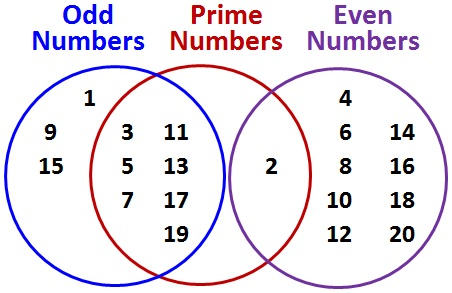© Ms. Garcia Math 7th Several students were asked about their favorite subjects in school GR33 answersAnswer the questions based on the Venn Diagram 1) How many odd numbers are there between 1 and 20? 2) How many even numbers are there between 1 and 20? 10 10 Answer Answer 3) How many prime numbers are there between 1 and 20? 4) How many numbers are odd numbers and prime numbers? 7 10 Answer Answer 5) How many numbers are even numbers and prime numbers? 6) How many numbers are not prime numbers? 17 12 Answer Answer Name: ID: